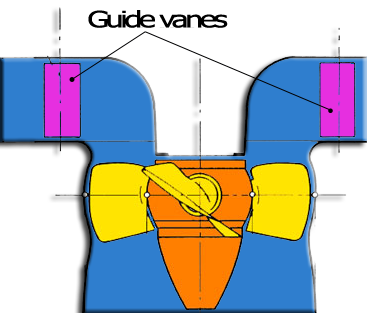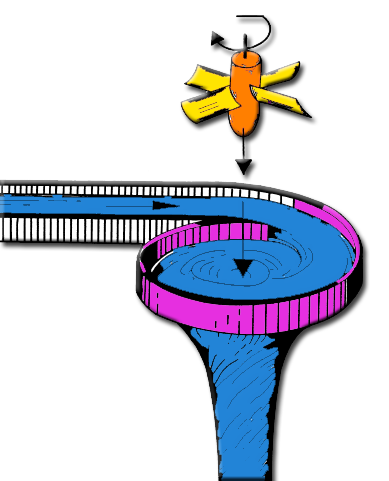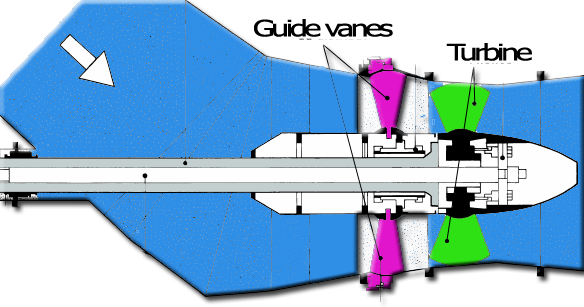Design: propeller turbine, screw, aerial, marine, turbine, tidal, wind, kaplan, foil, wings, 3D. Discover heliciel software: Modeling turbine kaplan in heliciel guide vanes draft tube turbine

kaplan hydraulic turbine energy capture 2/3 :guide vanes + turbineChapter Summary hydroelectric turbines::

Let's detail the operation of the propeller and guide vanes:
A turbine can only capture the kinetic energy of the fluid and therefore not directly captures the potential energy of the fluid height.Our hydraulic energy is composed of height energy, and kinetic energy, we need to convert the height energy into kinetic energy before passing through the turbine.

This is the role of guide vanes, which uses the pressure generated by the height to force the fluid through its vanes oriented to generate a vortex. The height energy (head load) is transformed into tangential velocity.It is important to differentiate the two components in the fluid velocity through the turbine: the axial velocity and tangential velocity::
• Axial velocity is the velocity in the direction parallel to the axis of turbine. The system throughput is given by the axial velocity multiplied by the area swept by the propeller section, also called section turbinated.Capture the kinetic energy (velocity) of a fluid consists in taking its speed, hence to slowing it down. If the propeller captured all the kinetic energy of the fluid, the fluid would not have velocity when leaving the turbine. This would imply that, deprived of all movements fluid would accumulate and clog the outlet of the turbine. So we must leave a little bit of kinetik power to allow the fluid circulation. This energy allowed to fluid, thus limiting the performance to a maximum : the Betz limit (see theory froude capture energy), If our velocity is only axial limit performance of our system is 0.6. This is the limit of performance for wind turbines or tidal turbines which we can not control the pressure upstream
• Débit (m3/sec) = Axial velocity(m/sec) X section swept by the propeller(m²).• The tangential velocity is the rotational speed of the vortex generated by the guide vanes or stator(sometimes combined with a volute)Unlike the axial velocity, the tangential velocity can be fully captured (stopped) . A tangential velocity fully captured, becomes zero, and leads to a general axial flow only.The guide vane or stator converts the potential energy of height(head load), in tangential velocity of rotation of the vortex . The quality of energy capture tangential velocity is optimum when the flow out of our propeller has lost all its tangential component.
We will remember: the energy of the height is converted to tangential velocity, which we can capture all of the energy and the volume flow, generates an axial velocity, which we can extract only 60% of the energy. To provides a maximum performance , a systemwill require transform the maximum hydraulic energy into tangential velocity, and that tangential velocity must be fully captured by the turbine. The quality of our system lies in the judicious combination of the tangential velocity and flow rate. The flow rate and collected heighttherefore the tangential velocity produced evolves irregularly depending on the season and weather. If we add to this that the speed of our generator must be steady and constant, we see the difficulty in designing an effective system.

Fortunately, to overcome these difficulties, setting organs of such as guide vanes, with its vanes and turbine blades adjustable, are used to control the combination of flow rate, tangential velocity and rotational speed of the turbine.Although these organs of settings control the blade pitch and the tangential velocity, we must calculate a inclination of the blade and a tangential velocity, to an optimum charge height, and a given flow rate of operation
The design method of the propeller turbine capture of energy, that we will propose in the rest of this article, will give the tangential velocity parameters to create with the guide vanes, according to the turbine optimized for a given flow rate. The load or pressure difference caused by the crossing of the turbine, giving the height of drop required to operate our turbine. The axial and tangential velocities at the turbine outlet, can be used respectively for sizing the draft tube and pipe sections and verify that the kinetic energy of the tangential speed, has been completely collected.

Video 3D Model turbine in héliciel software:

To facilitate this turbine design, we use the software HELICIEL, which has the advantage of automatically creating the optimum blade twist for a given operating point.
The database of profiles in software HELICIEL enable the design a 3D model of blade to igs format according to the selected profiles. low pressures calculated on the blades will be compared by the software, to cavitation pressure, depending on the depth of the propeller shaft. The goal is to make a complete study of the system in full autonomy, without tackling complex calculations of fluid mechanics. HELICIEL produce 3D shapes of propeller blades that you can assemble in the mechanical assembly. Software for industrial design, style, autocad or solidworks, will still be necessary for mechanical design
Next: The design method of turbinee

Chapter Summary hydroelectric turbines::